2018-12-06 16:22:35 zwqjoy 阅读数 8545
• ###### Kubernetes 实战系列

Kubernetes（KS8） 实战系列教程，该课程将对Kubernetes的Node、Pod、Replication Controller、Service等基本核心概念进行讲解，这些组件共同构成了Kubernetes的系统框架和计算模型。通过对它们进行灵活的组合，用户就可以快速、方便地对容器集群进行配置、创建和管理。

9532 人正在学习 去看看 CSDN讲师

# 背景介绍

Confusion Matrix -> Lift，Gain，ROC。

ROC -> AUC，KS -> GINI。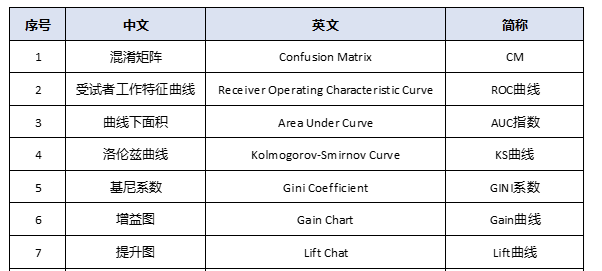1. 帮主是ROC曲线。

2. 副帮主是KS曲线，AUC面积

3. AUC养了一个小弟，叫GINI系数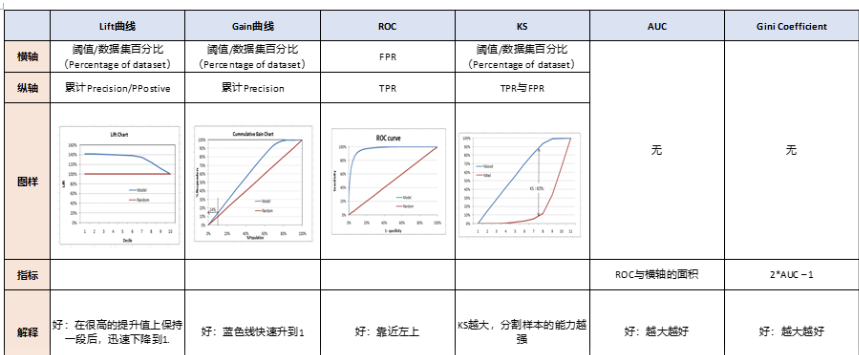# 二 KS曲线

KS曲线其实数据来源和本质和ROC曲线是一致的，只是ROC曲线是把真正率和假正率当作横纵轴，而K-S曲线是把真正率和假正率都当作是纵轴，横轴则由选定的阈值来充当。

KS(Kolmogorov-Smirnov)：KS用于模型风险区分能力进行评估，指标衡量的是好坏样本累计分部之间的差值。好坏样本累计差异越大，KS指标越大，那么模型的风险区分能力越强。

KS的计算步骤如下：
1. 计算每个评分区间的好坏账户数。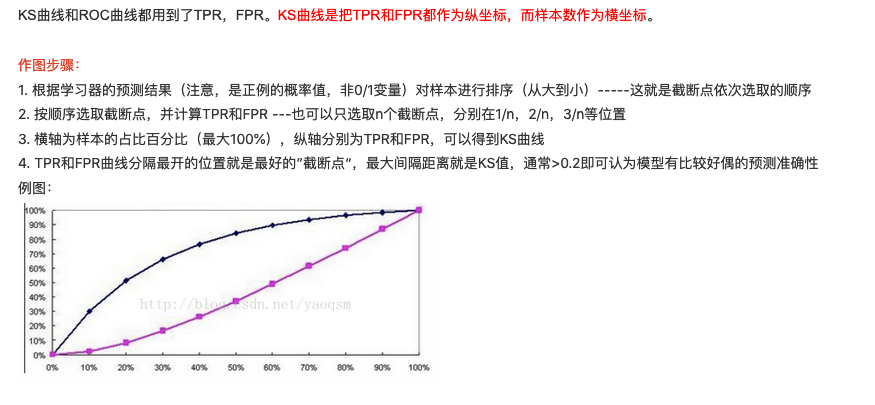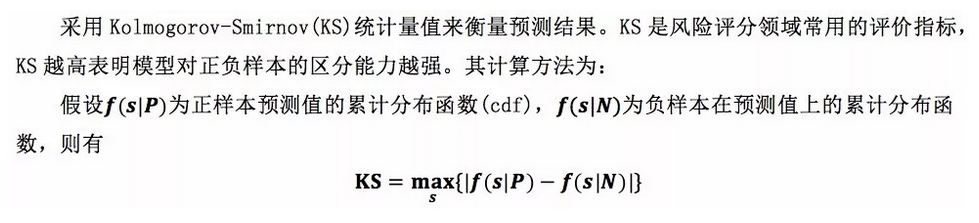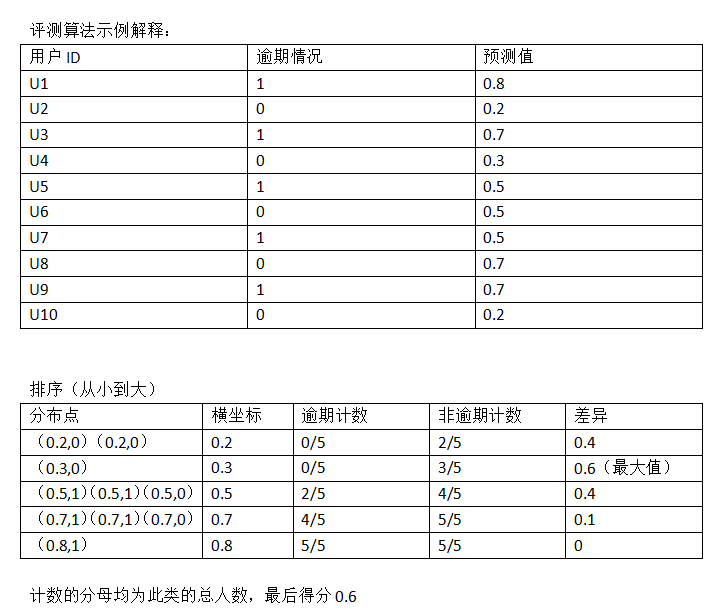https://blog.csdn.net/sinat_30316741/article/details/80018932

ROC值一般在0.5-1.0之间。值越大表示模型判断准确性越高，即越接近1越好。ROC=0.5表示模型的预测能力与随机结果没有差别。
KS值表示了模型将+和-区分开来的能力。值越大，模型的预测准确性越好。一般，KS>0.2即可认为模型有比较好的预测准确性。
KS值一般是很难达到0.6的，在0.2~0.6之间都不错。一般如果是如果负样本对业务影响极大，那么区分度肯定就很重要，此时K-S比AUC更合适用作模型评估，如果没什么特别的影响，那么用AUC就很好了。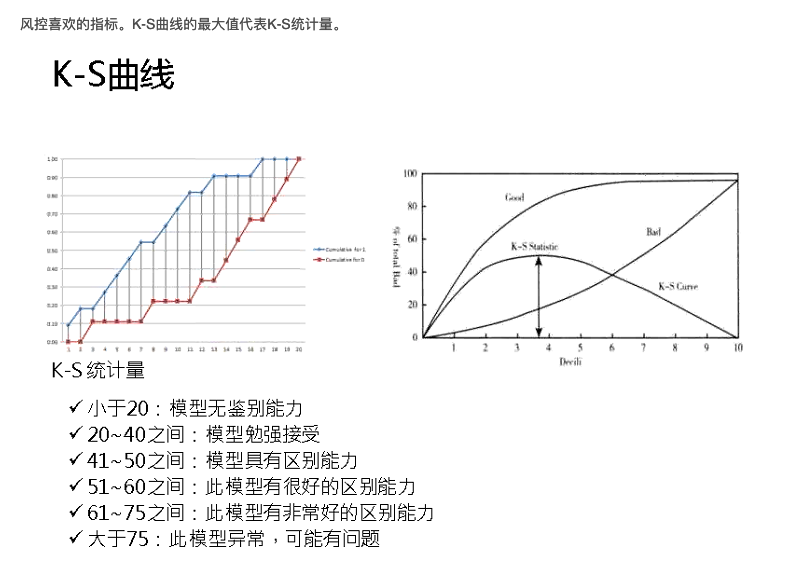# 三 GINI系数

·GINI系数:也是用于模型风险区分能力进行评估。
GINI统计值衡量坏账户数在好账户数上的的累积分布与随机分布曲线之间的面积，好账户与坏账户分布之间的差异越大，GINI指标越高，表明模型的风险区分能力越强。

GINI系数的计算步骤如下：
1. 计算每个评分区间的好坏账户数。
4. 计算出图中阴影部分面积，阴影面积占直角三角形ABC面积的百分比，即为GINI系数。

# 四 Lift , Gain

Lift图衡量的是，与不利用模型相比，模型的预测能力“变好”了多少，lift(提升指数)越大，模型的运行效果越好。
Gain图是描述整体精准度的指标。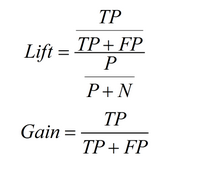1. 根据学习器的预测结果（注意，是正例的概率值，非0/1变量）对样本进行排序（从大到小）-----这就是截断点依次选取的顺序
2. 按顺序选取截断点，并计算Lift和Gain ---也可以只选取n个截断点，分别在1/n，2/n，3/n等位置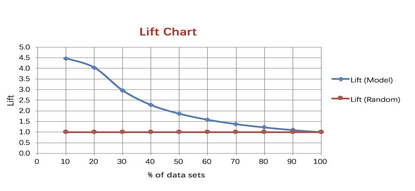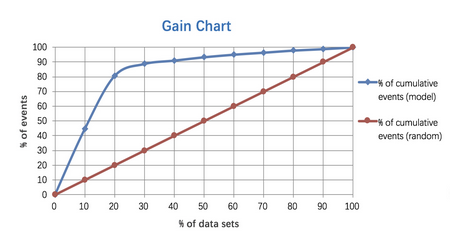# 五 模型稳定度指标PSI

PSI = sum（（实际占比-预期占比）* ln（实际占比/预期占比））

PS：除了按概率值大小等距十等分外，还可以对概率排序后按数量十等分，两种方法计算得到的psi可能有所区别但数值相差不大。

ks 曲线 机器学习 相关内容

2017-10-24 21:24:51 yaoqsm 阅读数 15718
• ###### Kubernetes 实战系列

Kubernetes（KS8） 实战系列教程，该课程将对Kubernetes的Node、Pod、Replication Controller、Service等基本核心概念进行讲解，这些组件共同构成了Kubernetes的系统框架和计算模型。通过对它们进行灵活的组合，用户就可以快速、方便地对容器集群进行配置、创建和管理。

9532 人正在学习 去看看 CSDN讲师ROC曲线是评判一个模型好坏的标准，有两个值要知道，FPR（假正率）和TPR（真正率），ROC曲线就是以这两个值为坐标轴画的。比如逻辑回归得到的结果是概率，那么就要取阈值来划分正负，这时候，每划一个阈值，就会产生一组FPR和TPR的值，然后把这组值画成坐标轴上的一个点，这样，当选取多组阈值后，就形成了ROC曲线（每次选取一个不同的阈值，我们就可以得到一组FPR和TPR，即ROC曲线上的一点），AUC值就是ROC曲线下方的面积。图中画出的AUC=0.810就是这个模型能得出的最好的AUC值，其对应的阈值也是最好的划分。但是最好的阈值是不能通过这个图知道的，要通过KS曲线得出。

KS曲线的纵轴是表示TPR和FPR的值，就是这两个值可以同时在一个纵轴上体现，横轴就是阈值，，然后在两条曲线分隔最开的地方，对应的就是最好的阈值，也是该模型最好的AUC值，就比如是上图的AUC=0.810，下图中，一条曲线是FPR，一条是TPRks 曲线 机器学习 相关内容

2018-03-19 16:43:28 cymy001 阅读数 5751
• ###### Kubernetes 实战系列

Kubernetes（KS8） 实战系列教程，该课程将对Kubernetes的Node、Pod、Replication Controller、Service等基本核心概念进行讲解，这些组件共同构成了Kubernetes的系统框架和计算模型。通过对它们进行灵活的组合，用户就可以快速、方便地对容器集群进行配置、创建和管理。

9532 人正在学习 去看看 CSDN讲师

## ROC曲线与AUC

ROC曲线下方的面积是AUC，AUC表示对于随机选择的正类别样本确实为正类别，以及随机选择的负类别样本为正类别，分类器更确信前者的概率。

http://blog.csdn.net/cymy001/article/details/79366754
Python机器学习库sklearn分类问题的模型评估API参考：
http://blog.csdn.net/cymy001/article/details/79425233

import numpy as np
import matplotlib.pyplot as plt
from itertools import cycle
from sklearn import datasets
from sklearn.model_selection import train_test_split
from sklearn.preprocessing import label_binarize

# Import some data to play with
X = iris.data   #(150,4)的二维numpy数组<class 'numpy.ndarray'>
y = iris.target   #(150,)的一维numpy数组标签<class 'numpy.ndarray'>

# Binarize the output
#对类别标签也进行了二值化处理[0 1 2]==>[[1 0 0],[0 1 0],[0 0 1]]
n_classes = y.shape   #有多少个不同的类别

# Add noisy features to make the problem harder
random_state = np.random.RandomState(0)
n_samples, n_features = X.shape   #样本数150，特征数4
X = np.c_[X, random_state.randn(n_samples, 200 * n_features)]
#np.c_将多个对象连接到第二个数轴上,增加了800维的特征噪声
#np.random.randn(维度大小参数)从标准正态分布中返回一个或多个样本值
#这里利用随机种子进行改造np.random.RamdonState(0).randn
#np.random.rand(维度大小参数)随机样本位于[0, 1)中

# shuffle and split training and test sets
X_train, X_test, y_train, y_test = train_test_split(X, y, test_size=.5, random_state=0)   #数据集切分

#### sklearn库API——decision_function(ROC阈值)

%matplotlib inline
import matplotlib.pyplot as plt
from sklearn.metrics import roc_curve, auc
from sklearn.multiclass import OneVsRestClassifier
from scipy import interp

# Learn to predict each class against the other
classifier = OneVsRestClassifier(svm.SVC(kernel='linear', probability=True, random_state=random_state))
y_score = classifier.fit(X_train, y_train).decision_function(X_test)
#decision_function对于SVC返回每个样本距离决策边界的距离
#decision_function对于LR返回预测样本的置信度分数——该样本与超平面的有符号距离

# Compute ROC curve and ROC area for each class
fpr = dict()
tpr = dict()
roc_auc = dict()
for i in range(n_classes):
fpr[i], tpr[i], _ = roc_curve(y_test[:, i], y_score[:, i])
#y_test样例真实标签，y_score学习器预测的样例的概率
roc_auc[i] = auc(fpr[i], tpr[i])
#计算ROC曲线下方的面积，fpr假正例率数组(横坐标)，tpr真正例率数组(纵坐标）

#Plot of a ROC curve for a specific class
plt.rcParams['figure.figsize']=(8,5)
plt.figure()
plt.plot(fpr, tpr, color='darkorange', label='ROC curve (area = %0.2f)' % roc_auc)
plt.plot([0, 1], [0, 1], color='navy', linestyle='--')

plt.xlim([0.0, 1.0])
plt.ylim([0.0, 1.05])
plt.xlabel('False Positive Rate')
plt.ylabel('True Positive Rate')
plt.legend(loc="lower right")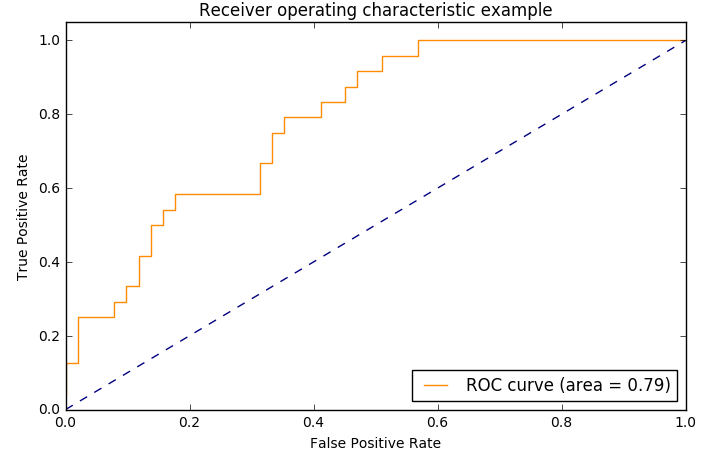##### Compute micro-average ROC curve and ROC area先计算每一类混淆矩阵，各类混淆矩阵作和除3，再算tpr和fpr
import numpy as np
fpr["micro"], tpr["micro"], _ = roc_curve(y_test.ravel(), y_score.ravel())   #ravel函数将矩阵展开成向量
roc_auc["micro"] = auc(fpr["micro"], tpr["micro"])
##### Compute macro-average ROC curve and ROC area先计算出每一类的tpr和fpr，再除3
import numpy as np
# First aggregate all false positive rates
all_fpr = np.unique(np.concatenate([fpr[i] for i in range(n_classes)]))   #np.concatenate将“特征维度相同数组”纵向拼接

# Then interpolate all ROC curves at this points
mean_tpr = np.zeros_like(all_fpr)   #np.zeros_like创建一个和参数all_fpr数组维度相同的全0数组
for i in range(n_classes):
mean_tpr += interp(all_fpr, fpr[i], tpr[i])
#interp一维线性插值，fpr和tpr是插值结点横纵坐标，all_fpr是已知中间节点横坐标(得到插值曲线后，求其纵坐标)
#https://docs.scipy.org/doc/numpy/reference/generated/numpy.interp.html#numpy.interp

# Finally average it and compute AUC
mean_tpr /= n_classes

fpr["macro"] = all_fpr
tpr["macro"] = mean_tpr
roc_auc["macro"] = auc(fpr["macro"], tpr["macro"])
%matplotlib inline
import numpy as np
import matplotlib.pyplot as plt
from itertools import cycle
from sklearn.metrics import roc_curve, auc

# Plot all ROC curves
plt.rcParams['figure.figsize']=(8,5)
plt.figure()
plt.plot(fpr["micro"], tpr["micro"], label='micro-average ROC curve (area = {0:0.2f})' ''.format(roc_auc["micro"]),
color='deeppink', linestyle=':')

plt.plot(fpr["macro"], tpr["macro"], label='macro-average ROC curve (area = {0:0.2f})' ''.format(roc_auc["macro"]),
color='navy', linestyle=':')

colors = cycle(['aqua', 'darkorange', 'cornflowerblue'])   #python3里的无穷循环器
for i, color in zip(range(n_classes), colors):
plt.plot(fpr[i], tpr[i], color=color, label='ROC curve of class {0} (area = {1:0.2f})' ''.format(i, roc_auc[i]))

plt.plot([0, 1], [0, 1], 'k--')
plt.xlim([0.0, 1.0])
plt.ylim([0.0, 1.05])
plt.xlabel('False Positive Rate')
plt.ylabel('True Positive Rate')
plt.title('Some extension of Receiver operating characteristic to multi-class')
plt.legend(loc="lower right")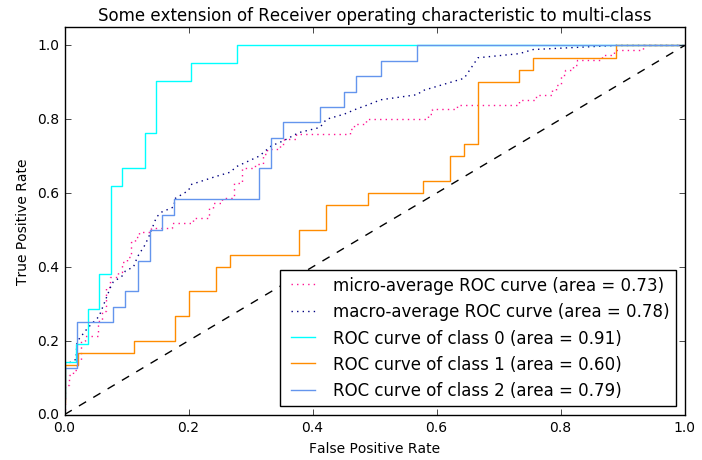#### 带交叉验证的ROC曲线

import numpy as np
from scipy import interp
import matplotlib.pyplot as plt
from itertools import cycle

from sklearn import svm, datasets
from sklearn.metrics import roc_curve, auc
from sklearn.model_selection import StratifiedKFold

# Data IO and generation

# Import some data to play with
X = iris.data
y = iris.target
X, y = X[y != 2], y[y != 2]    #去掉一类，下述交叉验证绘制ROC曲线只考虑二分类问题
n_samples, n_features = X.shape

random_state = np.random.RandomState(0)
X = np.c_[X, random_state.randn(n_samples, 200 * n_features)]

# Classification and ROC analysis

# Run classifier with cross-validation and plot ROC curves
cv = StratifiedKFold(n_splits=6)
classifier = svm.SVC(kernel='linear', probability=True, random_state=random_state)

tprs = []
aucs = []
mean_fpr = np.linspace(0, 1, 100)

i = 0
for train, test in cv.split(X, y):
# train和test是交叉验证分组索引
probas_ = classifier.fit(X[train], y[train]).predict_proba(X[test])   #predict_proba作用类似decision_function
# Compute ROC curve and area the curve
fpr, tpr, thresholds = roc_curve(y[test], probas_[:, 1])
tprs.append(interp(mean_fpr, fpr, tpr))   #根据每一折的fpr和tpr进行插值，得插值曲线后，对相同的mean_fpr求其对应插值曲线上的纵坐标
#interp一维线性插值，fpr和tpr是插值结点横纵坐标，mean_fpr是已知中间节点横坐标(得到插值曲线后，求其纵坐标)
#https://docs.scipy.org/doc/numpy/reference/generated/numpy.interp.html#numpy.interp
tprs[-1] = 0.0   #tprs有6个元素，每个元素是一个长度为100的array
roc_auc = auc(fpr, tpr)
aucs.append(roc_auc)   #aucs有6个auc的值，是交叉验证每一折的auc
plt.plot(fpr, tpr, alpha=0.3, label='ROC fold %d (AUC = %0.2f)' % (i, roc_auc))

i += 1
plt.plot([0, 1], [0, 1], linestyle='--', color='r', label='Luck', alpha=.8)

mean_tpr = np.mean(tprs, axis=0)   #将每一折交叉验证计算的tpr求和取平均(每个位置对应相同的fpr)
mean_tpr[-1] = 1.0
mean_auc = auc(mean_fpr, mean_tpr)
std_auc = np.std(aucs)
plt.plot(mean_fpr, mean_tpr, color='b', label=r'Mean ROC (AUC = %0.2f $\pm$ %0.2f)' % (mean_auc, std_auc), alpha=.8)

std_tpr = np.std(tprs, axis=0)
tprs_upper = np.minimum(mean_tpr + std_tpr, 1)
tprs_lower = np.maximum(mean_tpr - std_tpr, 0)
plt.fill_between(mean_fpr, tprs_lower, tprs_upper, color='grey', alpha=.2, label=r'$\pm$ 1 std. dev.')

plt.xlim([-0.05, 1.05])
plt.ylim([-0.05, 1.05])
plt.xlabel('False Positive Rate')
plt.ylabel('True Positive Rate')
plt.legend(loc="lower right")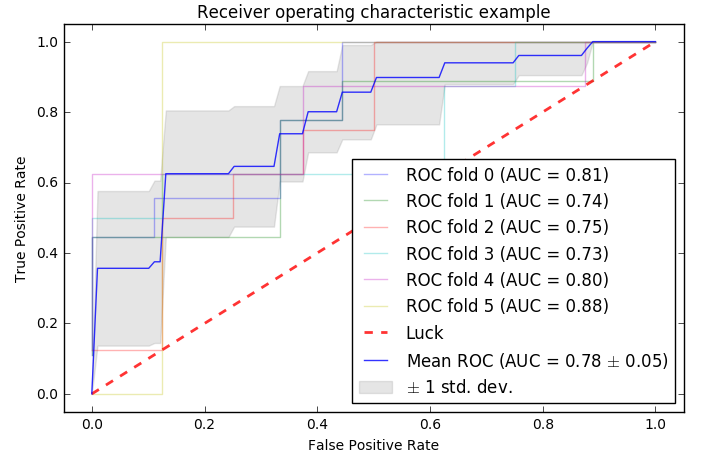## K-S曲线与KS值

K-S曲线是正样本洛伦兹曲线与负样本洛伦兹曲线的差值曲线，用来度量阳性与阴性分类区分程度的。K-S曲线的最高点(最大值)定义为KS值，KS值越大，模型的区分度越好。

（1）把Logistic Regression模型对样本的输出概率(predict_proba)从大到小排序$rank-threshold$$rank-threshold$
（2）计算阈值取每个概率时对应的TPR和FPR值
（3）以$\left(rank-threshold，TPR\right)$$(rank-threshold，TPR)$画正样本曲线，以$\left(rank-threshold，FPR\right)$$(rank-threshold，FPR)$画负样本曲线，就构成K-S曲线
（4）K-S曲线上的KS值，即$max\left(TPR-FPR\right)$$max(TPR-FPR)$，即两条曲线间的最大间隔距离。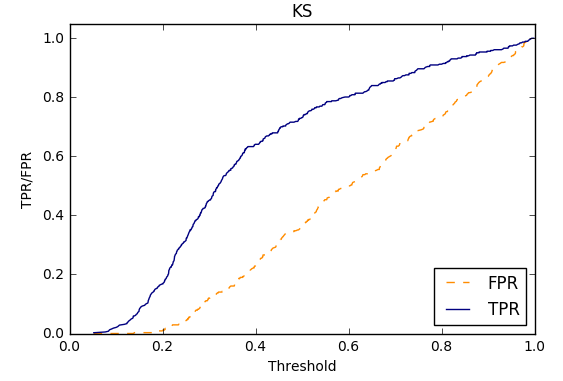$TPR-FPR$$TPR-FPR$最大时，即$\mathrm{\Delta }\left(TPR-FPR\right)=0$$\Delta(TPR-FPR)=0$，也就是ROC曲线上最优阈值满足的条件$\mathrm{\Delta }TPR=\mathrm{\Delta }FPR$$\Delta TPR=\Delta FPR$。通过，K-S曲线可以直观地找出模型中差异最大的一个分段，但K-S曲线不能反映出所有分段的效果。

ks 曲线 机器学习 相关内容

2019-06-20 12:04:09 weixin_31866177 阅读数 912
• ###### Kubernetes 实战系列

Kubernetes（KS8） 实战系列教程，该课程将对Kubernetes的Node、Pod、Replication Controller、Service等基本核心概念进行讲解，这些组件共同构成了Kubernetes的系统框架和计算模型。通过对它们进行灵活的组合，用户就可以快速、方便地对容器集群进行配置、创建和管理。

9532 人正在学习 去看看 CSDN讲师

## KS检验-风控角度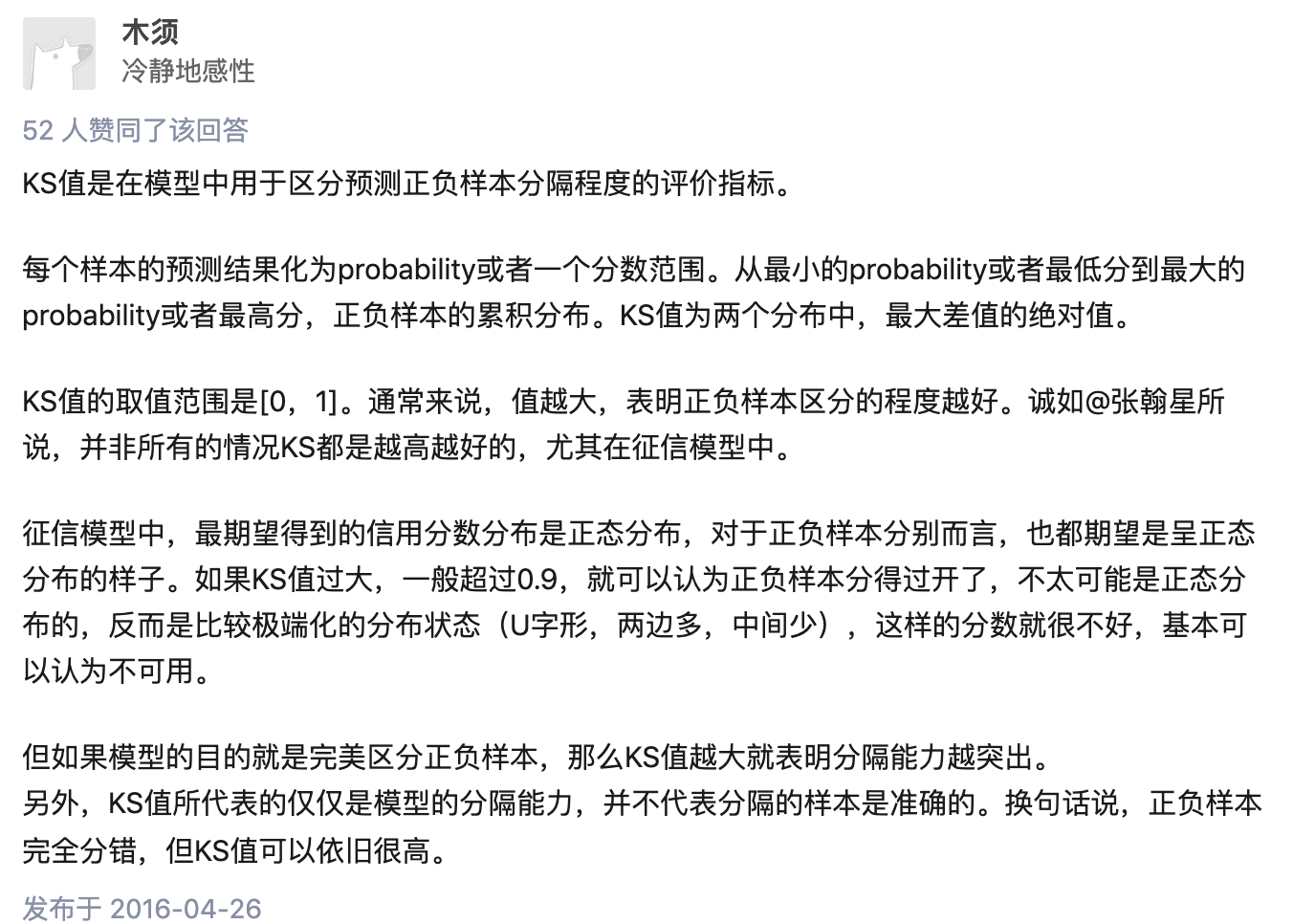def compute_ks(data1,data2):
df1 = DataFrame()
df1['pred'] = data2
df1['label'] = data1
# 按照样本为正样本的概率值升序排序，也即坏样本的概率从高到低排序
sorted_list = df1.sort_values(['pred'], ascending=[True])
# print(sorted_list)
"""
pred     label
17  0.055966    0.0
8   0.056266    0.0
14  0.063441    0.0
15  0.066217    0.0
0   0.070942    0.0
4   0.074102    0.0
3   0.087055    0.0
2   0.090387    0.0
18  0.092003    1.0
6   0.098286    0.0
16  0.105280    0.0
10  0.107256    0.0
12  0.123415    0.0
5   0.137829    0.0
7   0.139731    0.0
1   0.159905    0.0
13  0.180385    0.0
9   0.203199    0.0
11  0.217903    0.0
"""
total_good = sorted_list['label'].sum() # label为1的样本有多少个，真实为1的样本
# print(sorted_list['label'])
# print(total_good)
total_bad = sorted_list.shape - total_good # label为0的样本有多少个，真实为0的样本

max_ks = 0.0
good_count = 0.0
for index, row in sorted_list.iterrows(): #按照标签和每行拆开
# print(index)
# print('-'*5)
# print(row)
"""
index: 17
row:
pred     0.055966
label    0.000000
"""
if row['label'] == 0:
else:
good_count += 1
max_ks = max(max_ks, val)
return max_ks

def cal_auc(labels, preds):
"""
先排序，然后统计有多少正负样本对满足：正样本预测值>负样本预测值, 再除以总的正负样本对个数
复杂度 O(NlogN), N为样本数
"""
n_pos = sum(labels)
n_neg = len(labels) - n_pos
total_pair = n_pos * n_neg

labels_preds = zip(labels, preds)
labels_preds = sorted(labels_preds, key=lambda x: x)
accumulated_neg = 0
satisfied_pair = 0
for i in range(len(labels_preds)):
if labels_preds[i] == 1:
satisfied_pair += accumulated_neg
else:
accumulated_neg += 1

return satisfied_pair / float(total_pair)

def approximate_auc(labels, preds, n_bins=100):
"""
近似方法，将预测值分桶(n_bins)，对正负样本分别构建直方图，再统计满足条件的正负样本对
复杂度 O(N)
这种方法有什么缺点？怎么分桶？

"""
n_pos = sum(labels)
n_neg = len(labels) - n_pos
total_pair = n_pos * n_neg

pos_histogram = [0 for _ in range(n_bins)]
neg_histogram = [0 for _ in range(n_bins)]
bin_width = 1.0 / n_bins
for i in range(len(labels)):
nth_bin = int(preds[i] / bin_width)
if labels[i] == 1:
pos_histogram[nth_bin] += 1
else:
neg_histogram[nth_bin] += 1

accumulated_neg = 0
satisfied_pair = 0
for i in range(n_bins):
satisfied_pair += (pos_histogram[i] * accumulated_neg + pos_histogram[i] * neg_histogram[i] * 0.5)
accumulated_neg += neg_histogram[i]

return satisfied_pair / float(total_pair)

# official
def calc_ks(y_true, y_prob, n_bins=10):
percentile = np.linspace(0, 100, n_bins + 1).tolist()
bins = [np.percentile(y_prob, i) for i in percentile]
bins = bins - 0.01
bins[-1] = bins[-1] + 0.01
binids = np.digitize(y_prob, bins) - 1
y_1 = sum(y_true == 1)
y_0 = sum(y_true == 0)
bin_true = np.bincount(binids, weights=y_true, minlength=len(bins))
bin_total = np.bincount(binids, minlength=len(bins))
bin_false = bin_total - bin_true
true_pdf = bin_true / y_1
false_pdf = bin_false / y_0
true_cdf = np.cumsum(true_pdf)
false_cdf = np.cumsum(false_pdf)
ks_list = np.abs(true_cdf - false_cdf).tolist()
ks = max(ks_list)
return ks

# 在网上看其他人实现的方案，但是感觉是错的，和我们的问题可能有出入，
# 等之后再研究吧
from scipy.stats import ks_2samp
get_ks = lambda y_pred,y_true: ks_2samp(y_pred[y_true==1], y_pred[y_true!=1]).statistic
get_ks(x,y)
# mine
ks = ks_2samp(y, y_pred)
print("ks:",ks.statistic)

• FPRate的意义是所有真实类别为0的样本中，预测类别为1的比例。（好人为1）

f(good) 预测为负,真实为正除以所有真实正样本数。真正例率：

• TPRate的意义是所有真实类别为1的样本中，预测类别为1的比例。（坏人为0）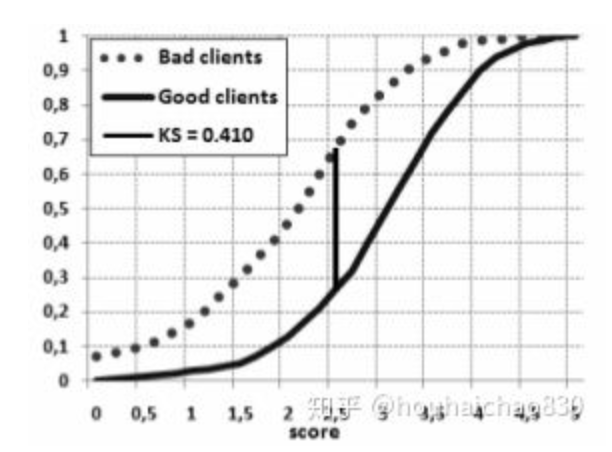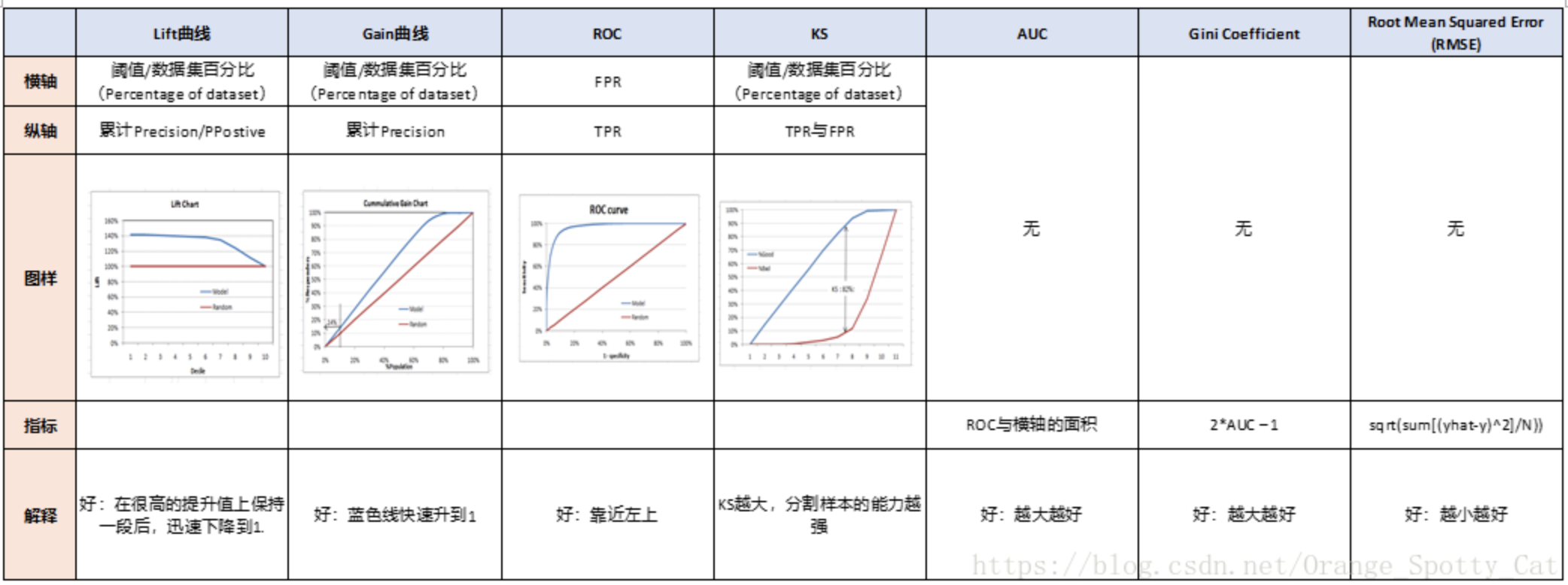def ks_2samp(data1, data2):
"""
Computes the Kolmogorov-Smirnov statistic on 2 samples.
This is a two-sided test for the null hypothesis that 2 independent samples
are drawn from the same continuous distribution.

data: 而为数组或dataframe，包括模型得分和真实的标签
score_col:一维数组或series，代表模型得分（一般为预测正类的概率）
class_col:一维数组或series，代表真实的标签（（0，1）or（-1，1））

ks：ks值
cdf_df:好坏人累积概率分布以及其差值gap

Parameters
----------
data1, data2 : sequence of 1-D ndarrays
two arrays of sample observations assumed to be drawn from a continuous
distribution, sample sizes can be different
Returns
-------
statistic : float
KS statistic
pvalue : float
two-tailed p-value
Notes
-----
This tests whether 2 samples are drawn from the same distribution. Note
that, like in the case of the one-sample K-S test, the distribution is
assumed to be continuous.
This is the two-sided test, one-sided tests are not implemented.
The test uses the two-sided asymptotic Kolmogorov-Smirnov distribution.
If the K-S statistic is small or the p-value is high, then we cannot
reject the hypothesis that the distributions of the two samples
are the same.
Examples
--------
>>> from scipy import stats
>>> np.random.seed(12345678)  #fix random seed to get the same result
>>> n1 = 200  # size of first sample
>>> n2 = 300  # size of second sample
For a different distribution, we can reject the null hypothesis since the
pvalue is below 1%:
>>> rvs1 = stats.norm.rvs(size=n1, loc=0., scale=1)
>>> rvs2 = stats.norm.rvs(size=n2, loc=0.5, scale=1.5)
>>> stats.ks_2samp(rvs1, rvs2)
(0.20833333333333337, 4.6674975515806989e-005)
For a slightly different distribution, we cannot reject the null hypothesis
at a 10% or lower alpha since the p-value at 0.144 is higher than 10%
>>> rvs3 = stats.norm.rvs(size=n2, loc=0.01, scale=1.0)
>>> stats.ks_2samp(rvs1, rvs3)
(0.10333333333333333, 0.14498781825751686)
For an identical distribution, we cannot reject the null hypothesis since
the p-value is high, 41%:
>>> rvs4 = stats.norm.rvs(size=n2, loc=0.0, scale=1.0)
>>> stats.ks_2samp(rvs1, rvs4)
(0.07999999999999996, 0.41126949729859719)
"""
data1 = np.sort(data1)
data2 = np.sort(data2)
n1 = data1.shape
n2 = data2.shape
data_all = np.concatenate([data1, data2])
cdf1 = np.searchsorted(data1, data_all, side='right') / (1.0*n1)
cdf2 = np.searchsorted(data2, data_all, side='right') / (1.0*n2)
d = np.max(np.absolute(cdf1 - cdf2))
# Note: d absolute not signed distance
en = np.sqrt(n1 * n2 / float(n1 + n2))
try:
prob = distributions.kstwobign.sf((en + 0.12 + 0.11 / en) * d)
except:
prob = 1.0

return Ks_2sampResult(d, prob)

houhaichao830

4.4.2分类模型评判指标（四） - ROC，AUC，GINI，KS，Lift，Gain，MSE总结

auc和ks是强相关的指标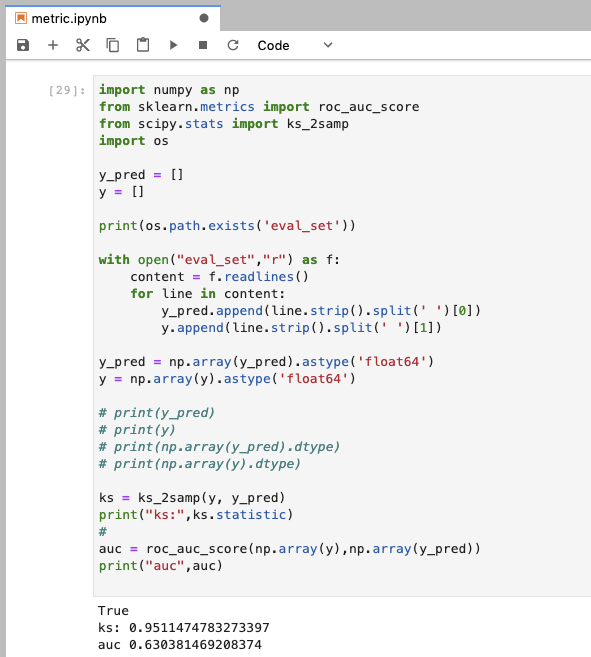astype转成一样的浮点数才可以进行减法操作！！！要dtype查看，之前这里报错辽~?

ks 曲线 机器学习 相关内容

2018-10-03 17:47:08 aa_JamesJones 阅读数 1491
• ###### Kubernetes 实战系列

Kubernetes（KS8） 实战系列教程，该课程将对Kubernetes的Node、Pod、Replication Controller、Service等基本核心概念进行讲解，这些组件共同构成了Kubernetes的系统框架和计算模型。通过对它们进行灵活的组合，用户就可以快速、方便地对容器集群进行配置、创建和管理。

9532 人正在学习 去看看 CSDN讲师

1. 混淆矩阵---确定截断点后，评价学习器性能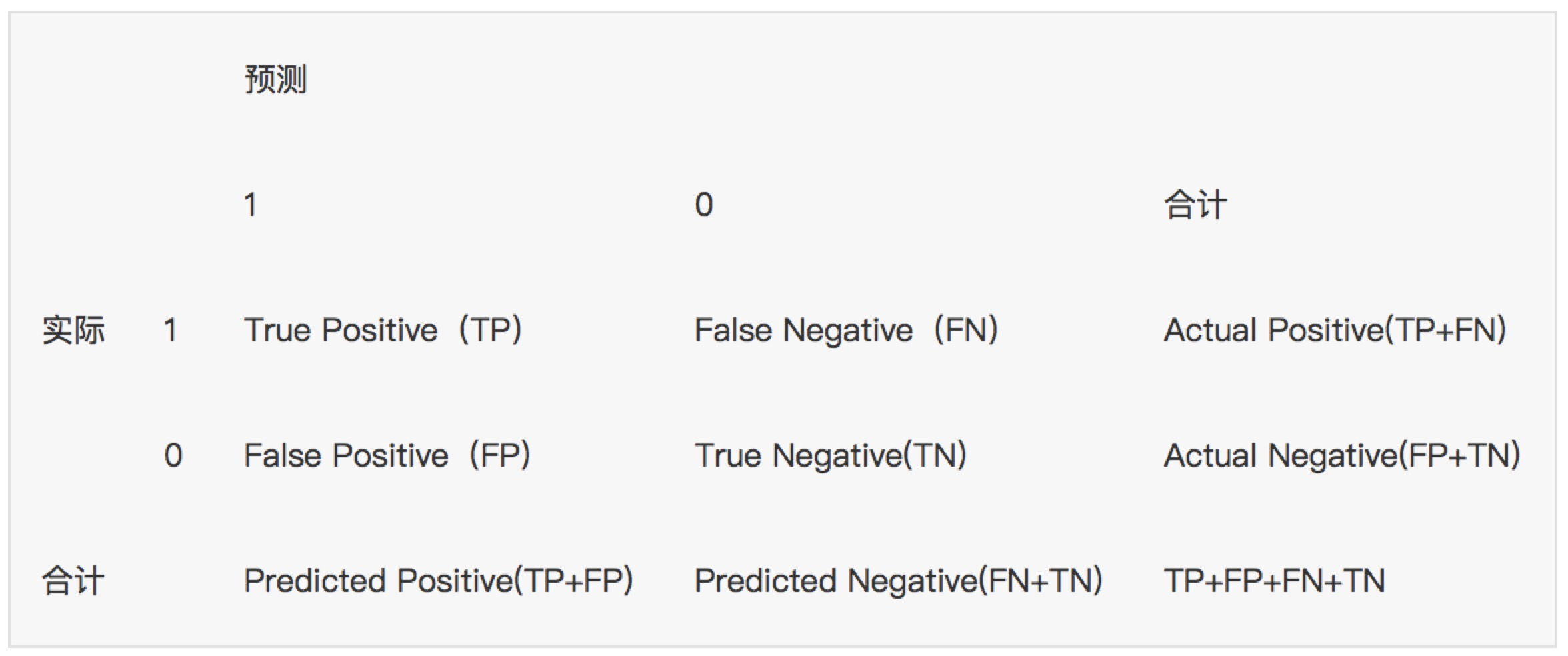TP（实际为正预测为正），FP（实际为负但预测为正），FN（实际为正但预测为负），TN（实际为负预测为负）

Accuracy=(TP+TN) / (TP+FP+FN+TN)

NPV=正确预测到的负例数/实际负例总数=TN / (TN+FN)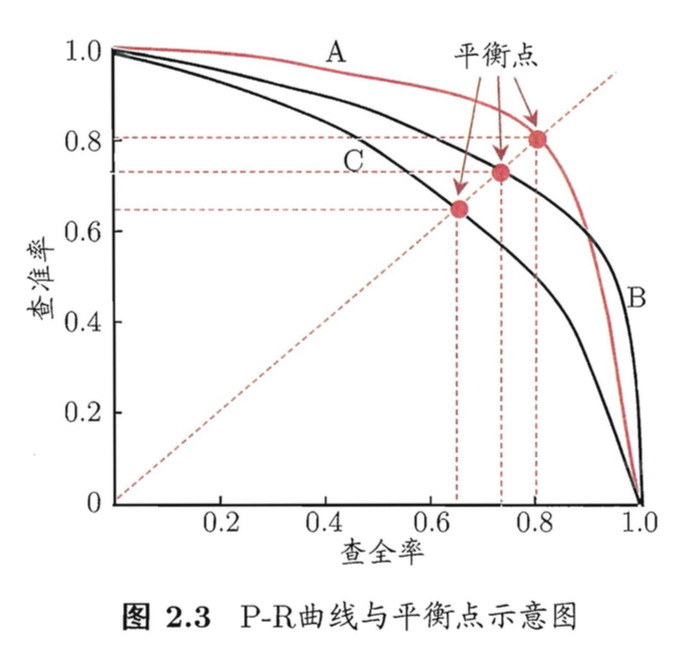2. F1-score---查准率和查全率的加权调和平均数

（1）当认为查准率和查全率一样重要时，权重相同时：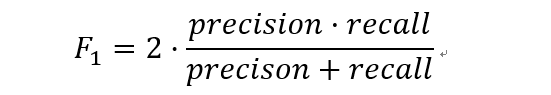（2）当查准率和查全率的重要性不同时，即权重不同时：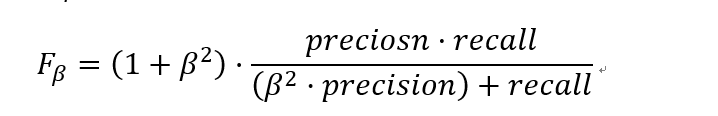1. β=1，查全率的权重=查准率的权重，就是F1

2. β>1，查全率的权重>查准率的权重

3. β<1，查全率的权重<查准率的权重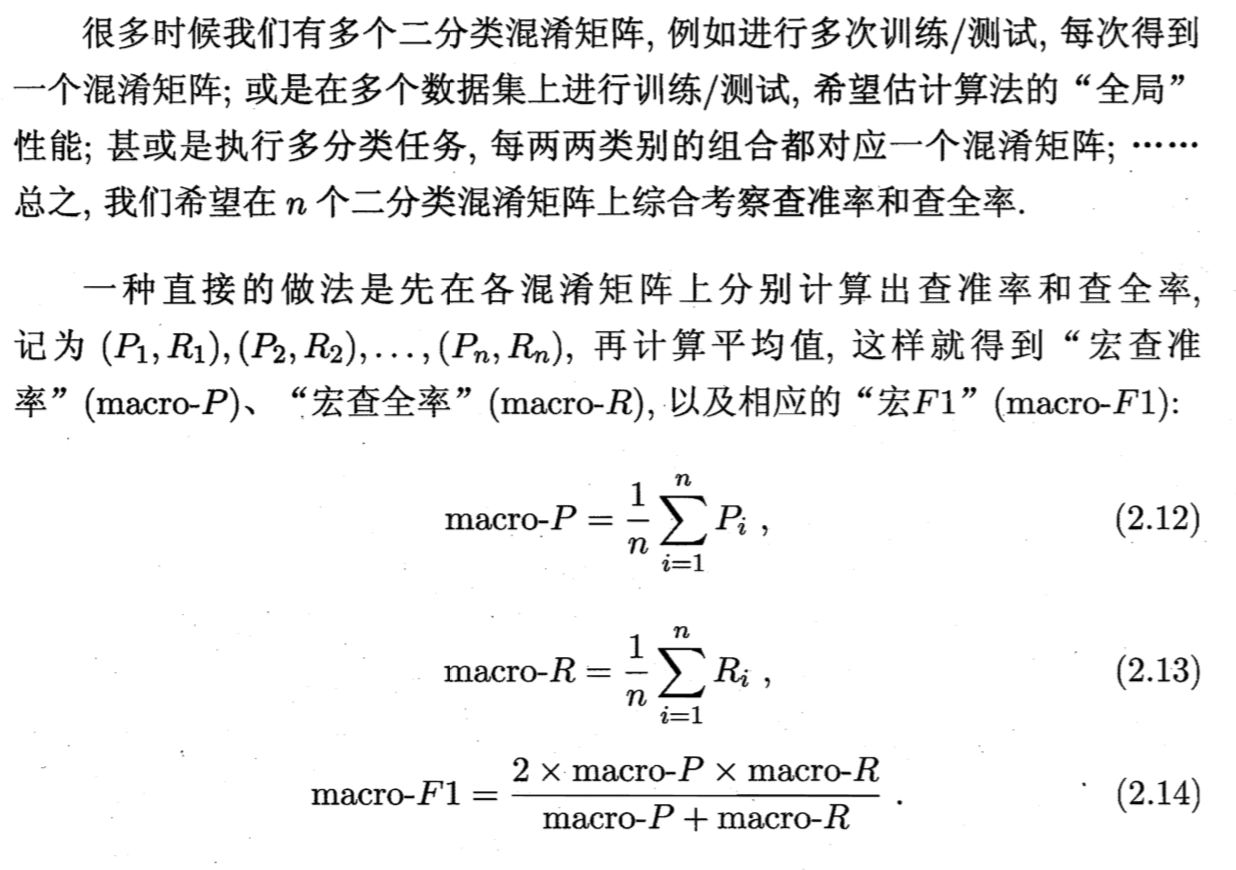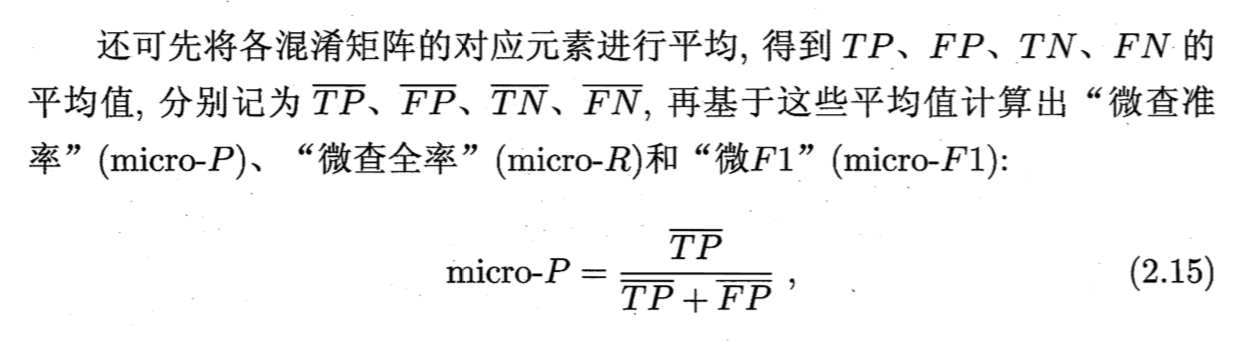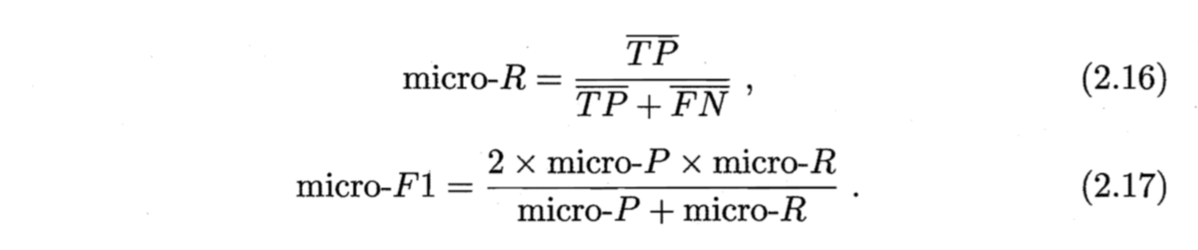3. ROC曲线， AUC---评价学习器性能，检验分类器对客户进行正确排序的能力

ROC曲线描绘的是不同的截断点时，并以FPR和TPR为横纵坐标轴，描述随着截断点的变小，TPR随着FPR的变化。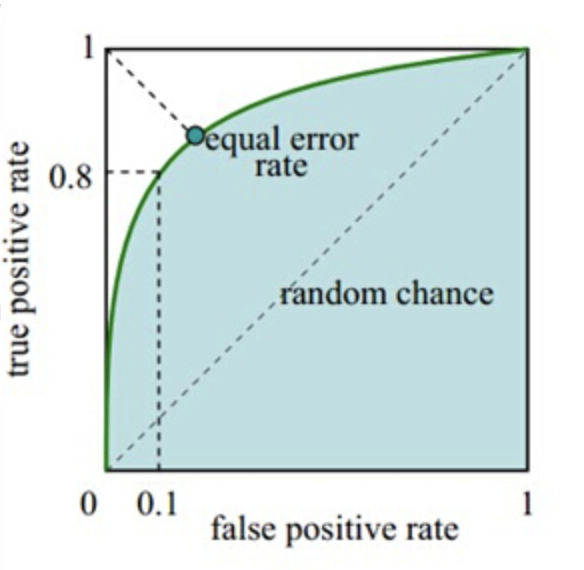1. 根据学习器的预测结果（注意，是正例的概率值，非0/1变量）对样本进行排序（从大到小）----- 这就是截断点（阈值threshold）依次选取的顺序

2. 按顺序选取截断点，并计算TPR和FPR（也可以只选取n个截断点）

3. 连接所有的点（TPR，FPR）即为ROC图

1. 一个ROC曲线完全”包住“另一个ROC曲线--->第一个学习器效果更好

2. 两个ROC曲线相交--->利用ROC曲线下的面积（AUC，area under ROC curve，是一个数值)进行比较

AUC：ROC曲线下的面积，由于ROC曲线一般都处于y=x这条直线的上方，所以AUC的取值范围在0.5和1之间。AUC作为数值可以直观的评价分类器的好坏，值越大越好。

AUC表示，随机抽取一个正样本和一个负样本，分类器正确给出正样本的score（预测样本是正例的概率）高于负样本的概率。在所有标注样本中，正样本共T个，负样本共F个，如果随机抽一个正样本和负样本，共有T*F个pair，其中如果有s个pair的正负样本pair满足于正样本的score高于负样本（权重为1），v个pair正负样本score相同（权重为0.5），则auc就等于(s+0.5v)/(T*F)。

4. KS曲线，KS值---学习器将正例和反例分开的能力，确定最好的“截断点”

KS曲线和ROC曲线都用到了TPR，FPR。KS曲线是把TPR和FPR都作为纵坐标，而阈值作为横坐标。

1. 根据学习器的预测结果（注意，是正例的概率值，非0/1变量）对样本进行排序（从大到小）-----这就是截断点依次选取的顺序

2. 按顺序选取截断点，并计算TPR和FPR（也可以只选取n个截断点）

3. 横轴为样本的占比百分比（最大100%），纵轴分别为TPR和FPR，可以得到KS曲线

4. TPR和FPR曲线分隔最开的位置就是最好的”截断点“，最大间隔距离就是KS值，通常>0.2即可认为模型有比较好的预测准确性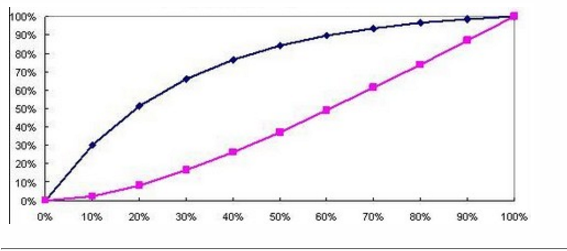KS曲线的纵轴是表示TPR和FPR的值，就是这两个值分别通过两条曲线来体现，横轴就是阈值（把0-1之间等分N份，等分点为阈值），然后在两条曲线分隔最开的地方，对应的就是最好的阈值，也是该模型最大的KS值。KS值的取值范围是[0，1] ，KS值越大，表示模型能够将正、负客户区分开的程度越大。

https://blog.csdn.net/yaoqsm/article/details/78334920

http://alexkong.net/2013/06/introduction-to-auc-and-roc/

ks 曲线 机器学习 相关内容